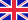•# APPLYING REGRESSION & CORRELAT

Autor: Jeremy Miles

This book takes a fresh look at applying regression analysis in the behavioural sciences by introducing the reader to regression analysis through a simple model-building approach.

The authors start with the basics and begin by re-visiting... Viac o knihe

Na objednávku, dodanie 2-4 týždne

93.10 €

bežná cena: 105.80 €

## O knihe

This book takes a fresh look at applying regression analysis in the behavioural sciences by introducing the reader to regression analysis through a simple model-building approach.

The authors start with the basics and begin by re-visiting the mean, and the standard deviation, with which most readers will already be familiar, and show that they can be thought of a least squares model. The book then shows that this least squares model is actually a special case of a regression analysis and can be extended to deal with first one, and then more than one independent variable.

Extending the model from the mean to a regression analysis provides a powerful, but simple, way of thinking about what students believe are the more complex aspects of regression analysis.

The authors gradually extend the model to include aspects of regression analysis such as non-linear regression, logistic regression, and moderator and mediator analysis. These approaches are often presented in terms that are too mathematical for non-statistically inclined students to deal with.

Throughout the book maintains a conceptual, non-mathematical focus. Most equations are placed in an appendix, where a detailed explanation is given, to avoid disrupting the flow of the main text.

This book will be indispensable for anyone using regression and correlation from undergraduates doing projects to postgraduate and researchers.

• Vydavateľstvo: SAGE Publications Ltd
• Rok vydania: 2001
• Formát: Paperback
• Rozmer: 234 x 156 mm
• Jazyk: Anglický jazyk
• ISBN: 9780761962304

Generuje redakčný systém BUXUS CMS spoločnosti ui42.